Courses

# Worksheet Question - Algebraic Expressions Class 7 Notes | EduRev

## Class 7 : Worksheet Question - Algebraic Expressions Class 7 Notes | EduRev

The document Worksheet Question - Algebraic Expressions Class 7 Notes | EduRev is a part of the Class 7 Course Mathematics (Maths) Class 7.
All you need of Class 7 at this link: Class 7

Q.1. Simplify : (a) 2x – {5y – (x – 2y)}
(b) 5a – {3a – (2 – a) + 4}

Ans. (a) 3x + 7y

(b) a + 2

Q.2. Pallavi spends ₹x daily and saves ₹ y per day. What is her income after 3 weeks?

Ans. ₹21 (x + y)

Q.3. If P = – 10, find the value of P2 – 2P – 100.

Ans. 20

Q.4. If a + b = 6, then find the value of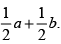Ans. 3

Q.5. From the sum of 3x – y + 11 and – y – 11, subtance 3x – y – 11.

Ans. –y + 11

Q.6. Write down the numerical coefficient in each of the following terms.

(i) xy (ii) –3xy (iii) 2p3 (iv) –5abc

Ans. (i) 1
(ii) –3
(iii) 2
(iv) –5

Q.7. Simplify the expression and find its value when a = 5 and b = –3. 2(a + ab) + 3 – ab

Ans. 2a2 + ab + 3, 38

Q.8. Add 4x2y, 8x2y and –2x2y.

Ans. 10x2y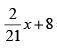= x + 6

Ans.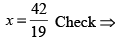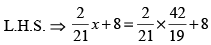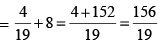R.H.S. =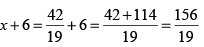= L.H.S. = R.H.S

Q.10. What should be added to a2 + ab + b2 to obtain 4ab + b2?

Ans. 2ab – b2

Q.11. The length of a rectangular field is 6m less than three times its breadth. Find the dimensions of the rectangle if its perimeter is 148 m.

Ans. Length = 54 m, Breadth = 20 m

Q.12. Collect like terms and simplify the expression : 12m2 – 9m + 5m – 4m2 – 7m + 10

Ans. 8 m– 11 m + 10

Q.13. What should be subtancted from a– 4a2 + 5a – 6 to obtain a2 – 2a + 1?

Ans. a– 5a+ 7a – 7

Q.14. In an isoceles triangle, the base angles are equal, the vertex angle is twice either the base angle. What are the degree measures of the angles of triangle?

Ans. Each of base angle = 45° Vertex angle = 90° 45°, 45° & 90°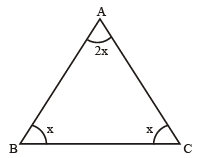Q.15. A bag contains 25 paise and so paise coins whose total values is ₹30. If the total number of 25 paise coins is four times that of 50 paise coins, find the number of each type of coins.

Ans. 50 Paise coins = 20

25 Paise coins = 80

Offer running on EduRev: Apply code STAYHOME200 to get INR 200 off on our premium plan EduRev Infinity!

,

,

,

,

,

,

,

,

,

,

,

,

,

,

,

,

,

,

,

,

,

;